# SBI PO Quantitative Aptitude Questions 2019 (Day-41) High Level New Pattern

Dear Aspirants, the most awaited notification of SBI PO – 2019 has been released. We all know that new pattern questions are introducing every year in the SBI PO exam. Further, the questions are getting tougher and beyond the level of the candidate’s expectations.

Our IBPS Guide is providing High-Level New Pattern Quantitative Aptitude Questions for SBI PO 2019 so the aspirants can practice it on a daily basis. These questions are framed by our skilled experts after understanding your needs thoroughly. Aspirants can practice these high-level questions daily to familiarize with the exact exam pattern. We wish that your rigorous preparation leads you to a successful target of becoming SBI PO.

#### “Be not afraid of growing slowly; be afraid only of standing still”

[WpProQuiz 6465]

Direction (1 – 5): Study the following data carefully and answer the questions:

There are five different mixture of milk of three types A, B and C. Bar graph given below shows the ratio of type A milk to type B milk in those five different mixtures.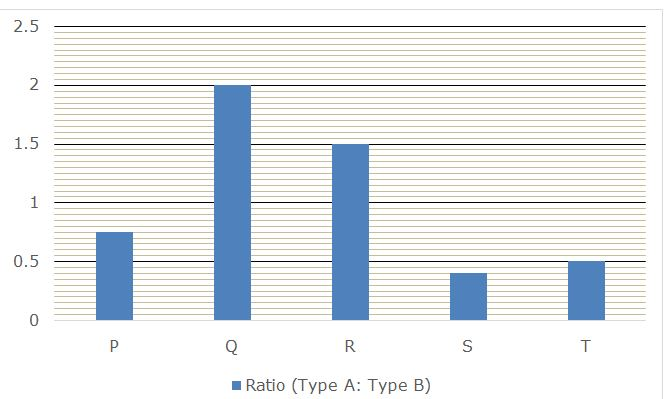Pie chart shows the distribution (degree) of type C milk in those five mixture and sum of the pie chart is 80 litres.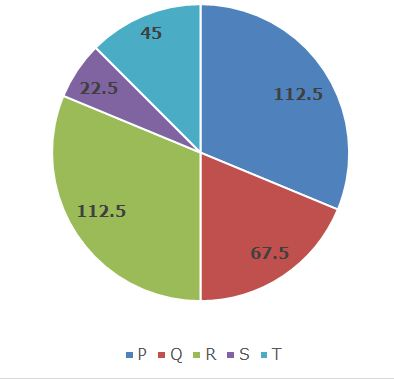1)If in mixture P, type C milk is 5 litres more than type B milk and ratio of type A to type C milk in the mixture Q is 4: 3. If mixture P and Q are mixed in the ratio 4: 3 to form mixture M, then out of total amount of mixture M, what per cent are type A milk?

a) 33(1/3)%

b) 25%

c) 26(2/3)%

d) 40%

e) None of these

2) If total quantity of mixture Q and R is 30 litres and 50 litres respectively. If these two mixtures are mixed in the ratio 6: 5 respectively to form mixture N, then what is the ratio of type A, type B and type C milk respectively in mixture N?

a) 8: 5: 9

b) 9: 3: 10

c) 6: 9: 7

d) 7: 4: 11

e) None of these

3) Mixture P and R are mixed completely to form mixture Z. Now, ratio of type A, type B and type C milk in the mixture Z becomes 9: 9: 5, then what is the difference between the amount of type A milk in mixture P and mixture R if amount of type B milk in mixture R is 30 litres?

a) 24 litres

b) 12 litres

c) 21 litres

d) 25 litres

e) None of these

4) Amount of type A milk in mixtures R and S are same and these two mixtures are mixed in the ratio 15: 22 to form mixture Z and amount of type C milk in mixture Z becomes (6/37). If the ratio of cost price of type A, type B and type C milk is 3: 2: 1 respectively and mixture Z is sold at the cost of type A milk, then what is the profit per cent earned?

a) 41.25%

b) 36.25%

c) 38.75%

d) 47.75%

e) None of these

5) Ratio of type B to type C milk in mixture S and T is 5: 1 and 1: 1 respectively. If these two mixtures are mixed in the ratio 4: 5 to form mixture M, what is the difference between type B and type C milk in 180 litres of mixture M?

a) 40 litres

b) 25 litres

c) 50 litres

d) 30 litres

e) None of these

Direction (6 – 10): Study the following data carefully and answer the questions:

There are two cities A and B in a state. A bird flies from city A to city B in the morning for its food and comes back to city A in the evening on five different days of a week starting from Monday to Friday.

Note 1: Flight speed (without wind) of bird from city A to city B in the morning is different on all the five days while its flight speed (without wind) from city B to city A in the evening is also different on all the five days.

2: Direction of wind remains same and is from city B to city A. Speed of wind in the morning is 50 km/h while the speed of wind in the evening is 40 km/h.

First pie chart given below shows the per cent distribution of flight speed (without wind) of bird from city A to city B in the morning on all the five days and sum of the pie chart is 1000 km/h.

Second pie chart given below shows the per cent distribution of flight speed (without wind) of bird from city B to city A in the evening on all the five days and sum of the pie chart is 800 km/h.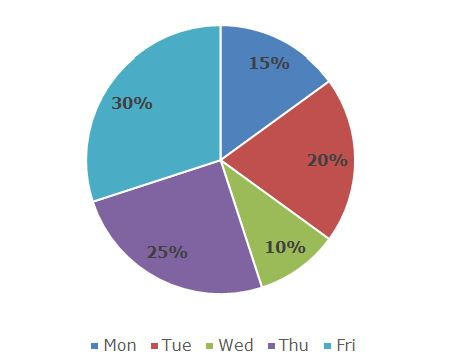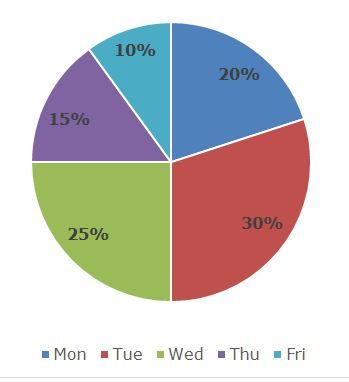6) If the distance between cities A and B is 270 km, then what is the difference between time taken by the bird to reach at city B from city A on Monday in morning and time taken by the bird reach at city A from city B on Friday in evening?

a) 36 minutes

b) 18 minutes

c) 45 minutes

d) 27 minutes

e) None of these

7) On Wednesday morning, the bird starts from city A towards city B and at the same time another bird with its flight speed (without wind) 120 km/h starts from city B towards city A. If difference between total distance travelled by both the bird before meeting is 90 km, then what is the distance between cities A and B?

a) 110 km

b) 220 km

c) 165 km

d) 198 km

e) None of these

8) If the bird starts flying from city A towards city B at 6:00 AM in the morning on Friday and search for food in the city. In the process of searching food it spends 7 hours 9 minutes there and comes back to the city A at 3:00 PM in the evening on the same day, then how far city B is from city A? Assume there is no other loss of time other than flight and searching.

a) 150 km

b) 120 km

c) 180 km

d) 135 km

e) None of these

9) If distance between cities A and B is 98 km and on Tuesday evening the speed of wind is increased by 20 km/h and the bird also increases its flight (without wind) speed while returning from B towards A. Now, time taken by bird to reach at A from B is decreased by 4.2 minutes than the calculated time, then what is the increment in the flight (without wind) speed of bird?

a) 40 km/h

b) 50 km/h

c) 25 km/h

d) 35 km/h

e) None of these

10) If on Thursday, bird flies from city A to city B in the morning but with its flight speed (without wind) 20% more than its original flight (without wind) speed and comes back to the original point in the evening on the same day with speed along the wind as mention in the charts, then how much less time the bird will take then the original time in its flight if cities A and B are 80 km apart?

a) 3.2 minutes

b) 5.4 minutes

c) 4.8 minutes

d) 3.6 minutes

e) None of these

Direction (1 – 5):

Ratio of type A to type B milk in mixture P = 0.75: 1 = 3: 4

Ratio of type A to type B milk in mixture Q = 2: 1

Ratio of type A to type B milk in mixture R = 1.5: 1 = 3: 2

Ratio of type A to type B milk in mixture S = 0.40: 1 = 2: 5

Ratio of type A to type B milk in mixture T = 0.5: 1 = 1: 2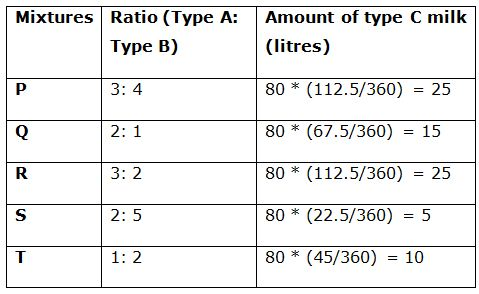Mixture P:

Type C milk = 25 litres

Type B milk = 25 – 5 = 20 litres

Type A milk = 20 * (3/4) = 15 litres

Part of type A milk = 15/(25 + 20 + 15) = 1/4

Mixture Q:

Type C milk = 15 litres

Type A milk = 15 * (4/3) = 20 litres

Type B milk = 20 * (1/2) = 10 litres

Part of type A milk = 20/(15 + 20 + 10) = 4/9

By the rule of allegation: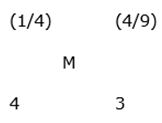[(4/9) – M]: [M – (1/4)] = 4: 3

(4/3) – 3M = 4M – 1

7M = 7/3

M = 1/3

Required per cent = (1/3) * 100 = 33(1/3)%

Mixture Q:

Type C milk = 15 litres

Type A milk = (30 – 15) * (2/3) = 10 litres

Type B milk = (30 – 15) * (1/3) = 5 litres

Part of type A milk = 10/(15 + 10 + 5) = 1/3

Part of type B milk = 5/(15 + 10 + 5) = 1/6

Mixture R:

Type C milk = 25 litres

Type A milk = (50 – 25) * (3/5) = 15 litres

Type B milk = (50 – 25) * (2/5) = 10 litres

Part of type A milk = 15/(25 + 15 + 10) = 3/10

Part of type B milk = 10/(25 + 15 + 10) = 1/5

By the rule of alligation: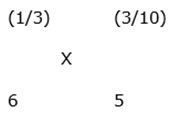[(3/10) – X]: [X – (1/3)] = 6: 5

(3/2) – 5X = 6X – 2

11X = 7/2

X = 7/22

By the rule of alligation: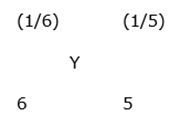[(1/5) – Y]: [Y – (1/6)] = 6: 5

1 – 5Y = 6Y – 1

11Y = 2

Y = 2/11 = 4/22

Required ratio = 7: 4: (22 – 7 – 4) = 7: 4: 11

Let amount of type A and type B milk in mixture P is 3p and 4p respectively.

Type B milk in mixture R = 30 litres

Type A milk in mixture R = 30 * (3/2) = 45 litres

Total quantity of mixture R = 45 + 30 + 25 = 100 litres

Amount of type A milk in mixture Z = (3p + 45)

Amount of type C milk in mixture Z = 25 + 25 = 50 litres

According to the question:

(3p + 45): 50 = 9: 5

15p + 225 = 450

15p = 225

p = 15

Hence, required difference = 3p ~ 45 = 45 – 45 = 0 litres

Let type A milk in mixture R and S is 6x each.

Type B milk in mixture R = 6x * (2/3) = 4x

Type B milk in mixture S = 6x * (5/2) = 15x

Part of type C milk in mixture R = 25/(10x + 25)

Part of type C milk in mixture S = 5/(21x + 5)

By the rule of alligation: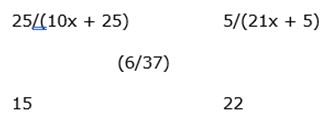[{5/(21x + 5)} – (6/37)]: [(6/37) –  {25/(10x + 25)}] = 15: 22

[110/(21x + 5)] – (132/37) = (90/37) – [375/(10x + 25)]

[110/(21x + 5)] + [375/(10x + 25] = 6

110(10x + 25) + 375(21x + 5) = 6(10x + 25)(21x + 5)

1100x + 2750 + 7875x + 1875 = 1260x2 + 3450x + 750

1260x2 – 5525x – 3875 = 0

252x2 – 1105x – 775 = 0

x = 5 and -155/252 (Not valid)

Amount of type A milk in mixture Z = 6x + 6x = 12x = 60

Amount of type B milk in mixture Z = 4x + 15x = 19x = 95

Amount of type C milk in mixture Z = 25 + 5 = 30

Cost price of mixture Z = (60 * 3) + (95 * 2) + (30 * 1) = 400

Selling price of mixture Z = (60 + 95 + 30) * 3 = 555

Required profit per cent = [(555 – 400)/400] * 100 = 38.75%

In 180 litres of mixture M:

Amount of mixture R = 180 * (4/9) = 80 litres

Ratio of type A, type B and type C milk in mixture R = 2: 5: 1

Amount of mixture S = 180 * (5/9) = 100 litres

Ratio of type A, type B and type C milk in mixture S = 1: 2: 2

Type B milk in mixture M = [80 * (5/8)] + [100 * (2/5)] = 50 + 40 = 90 litres

Type C milk in mixture M = [80 * (1/8)] + [100 * (2/5)] = 10 + 40 = 50 litres

Required difference = 90 – 50 = 40 litres

Directions (6 – 10):

Flight speed of bird (without wind) on Monday from A to B = 15% of 1000

= 150 km/h

Flight speed of bird (without wind) on Tuesday from A to B = 20% of 1000

= 200 km/h

Flight speed of bird (without wind) on Wednesday from A to B = 10% of 1000

= 100 km/h

Flight speed of bird (without wind) on Thursday from A to B = 25% of 1000

= 250 km/h

Flight speed of bird (without wind) on Friday from A to B = 30% of 1000

= 300 km/h

Flight speed of bird (without wind) on Monday from B to A = 20% of 800

= 160 km/h

Flight speed of bird (without wind) on Tuesday from B to A = 30% of 800

= 240 km/h

Flight speed of bird (without wind) on Wednesday from B to A = 25% of 800

= 200 km/h

Flight speed of bird (without wind) on Thursday from B to A = 15% of 800

= 120 km/h

Flight speed of bird (without wind) on Friday from B to A = 10% of 800

= 80 km/h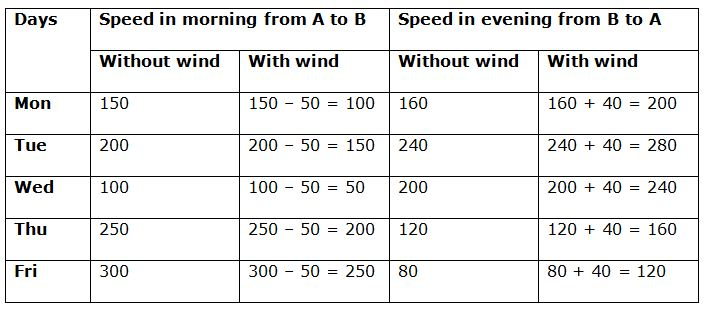Distance between cities A and B = 270 km

Time taken by the bird to reach at city B from city A on Monday in morning

= 270/100 = 2.7 hours

Time taken by the bird reach at city A from city B on Friday in evening

= 270/120 = 2.25 hours

Required difference = 2.7 – 2.25 = 0.45 hours = 27 minutes

Flight speed (without wind) of another bird on Wednesday morning = 120 km/h

Flight speed (with wind) of another bird on Wednesday morning = 120 + 50

= 170 km/h

Effective speed of both the birds = 50 + 170 = 220 km/h

Let both the birds meet after travelling for ‘t’ hours.

Required difference = 170t – 50t = 90

120t = 90

t = 0.75 hours

Distance between both the cities = 220 * 0.75 = 165 km

Time between 6:00 AM in the morning to 3:00 PM in the evening on the same day

= 9 hours = 540 minutes

Time taken in search of food = 7 hours 9 minutes = (7 * 60) + 9 = 429 minutes

Time taken in flight = 540 – 429 = 111 minutes

Let the distance between cities A and B is ‘D’ km.

According to the question:

(D/250) + (D/120) = (111/60)

(12D + 25D)/3000 = 111/60

37D = (111/60) * 3000

37D = 5550

D = 150

Hence, distance between cities A and B is 150 km.

Let increment in the flight speed of bird = ‘x’ km/h

Effective speed of bird on Tuesday evening while returning from B to A

= (280 + 20 + x) = (300 + x) km/h

Calculated time taken by bird = (98/280) * 60 = 21 minutes

Actual time taken by bird = (21 – 4.2) = (98 * 60)/(300 + x)

300 + x = 350

x = 50

Increment in the flight (without wind) speed of bird = x = 50 km/h

Changed flight speed (without wind) of the bird = 120% of 250 = 300 km/h

Changed flight speed (with wind) of the bird = 300 – 50 = 250 km/h

Original flight time of the bird = (80/200) + (80/160) = 0.4 + 0.5 = 0.9 hours

= 54 minutes

Original flight time of the bird = (80/250) + (80/160) = 0.32 + 0.5 = 0.82 hours

= 49.2 minutes

Required less time = 54 – 49.2 = 4.8 minutes